# Highlights

• New network architecture (SDNet) that factorizes 2D medical images into spatial anatomical factors and non-spatial modality factors;
• Various experiments to show that the learned representation is well-suited to a variety of image analysis tasks, including:
• semi-supervised segmentation
• multi-task segmentation and regression (e.g. Left Ventricular Volume estimation)
• image-to-image synthesis

# Introduction

Learning a decomposition of data into a spatial content factor and a non-spatial style factor has been a focus of recent research in computer vision[…].

This focus can be explained by the advantages of such a representation:

• Meaningful representation of the anatomy that can be generalized to any modality

• Suitable format for pooling information from various imaging modalities

# Methods

## Spatial Decomposition Network (SDNet)

The SDNet can be seen as an autoencoder that learns multiple factors, namely:

• $$s = f_A (x)$$: a multi-channel output of binary maps, representing the anatomical components
• $$z = f_M (x)$$: the $$Q(z \vert X)$$ multivariate Gaussian as in a standard VAE, representing the modality components

Other sub-networks are added to provide feedback over multiple tasks, with varying degrees of supervision:

• $$g$$: a self-supervised decoder that tries to reconstruct the input image from its $$s$$ and $$a$$ decomposition;
• $$h$$: a segmentor network that predicts the cardiac segmentation from $$s$$.
• When a ground truth is available for the current image, $$h$$ is trained using a standard Dice loss;
• Otherwise, the training is semi-supervised through a GAN-like adversarial loss, where a discriminator network tries to predict whether a segmentation mask was predicted by $$h$$ or comes from a pool of groundtruth segmentations.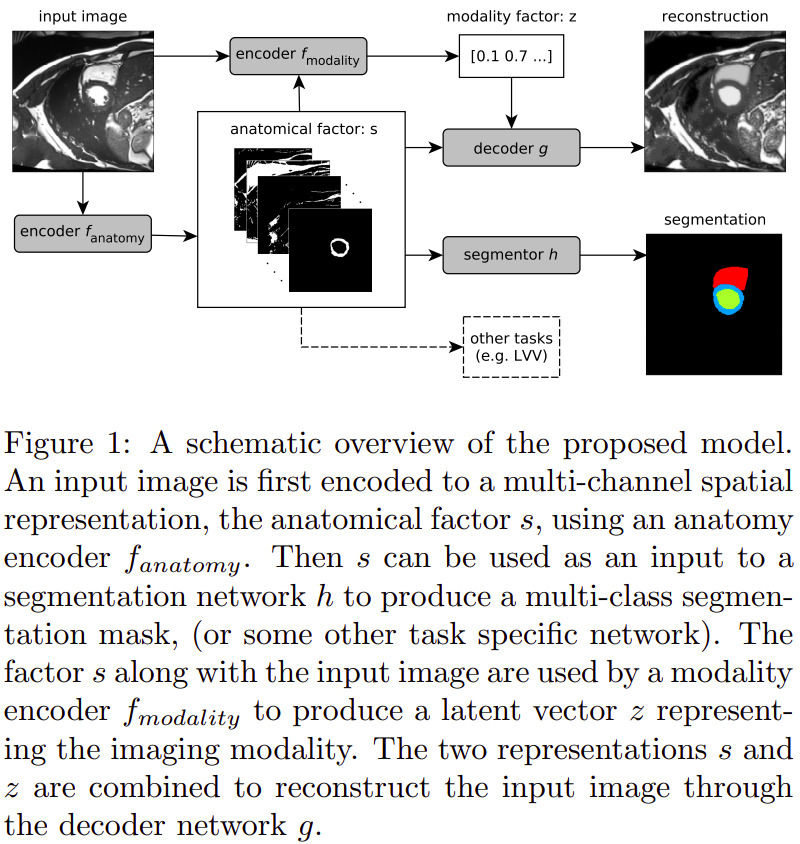The overall loss function is the following weighted sum (determined empirically):

$L = \lambda_1 L_{KL} + \lambda_2 L_{segm} + \lambda_3 L_{adv} + \lambda_4 L_{rec} + \lambda_5 L_{z_{rec}}$

where $$L_{KL}$$ and $$L_{rec}$$ make up the autoencoder’s self-supervision, and $$L_{segm}$$ and $$L_{rec}$$ correspond to the aforementioned segmentation supervision or semi-supervision. The additional factor is $$L_{z_{rec}}$$, which is enforces a modality factor reconstruction:

$L_{z_{rec}} = \mathbb{E}_{z,y} [\|z - f_{modality}(y, f_{anatomy}(y))\| _{1}]$

where $$y$$ is an image produced using a random $$z$$ sample. This is done to avoid a posterior collapse, where the decoder would ignore parts or the totality of the modality factor.

# Data

## Semi-supervised segmentation

• Training subset of the ACDC dataset:
• 1920 images with manual segmentations (ED and ES) and 23,530 images with no segmentations
• Edinburgh Imaging Facility QMRI: 26 healthy volunteers with around 30 cardiac phases each, acquired on a 3T scanner
• 241 images with manual segmentations (ED) and 8353 images with no segmentations

## Multimodal segmentation and modality transformation

• Data from the 2017 Multi-Modal Whole Heart Segmentation (MM-WHS) Challenge: 20 cardiac CT/CT angiography (CTA) volumes and 20 cardiac MRI volumes
• 3626 MR and 2580 CT images, all with manual segmentations of seven heart structures: myocardium, left atrium, left ventricle, right atrium, right ventricle, ascending aorta and pulmonary artery

## Modality estimation

• cine-MR and CP-BOLD images of 10 canines[…]. Two almost identical sequences with the only difference that CP-BOLD modulates pixel intensity with the level of oxygenation present in the tissue.

• 129 cine-MR and 264 CP-BOLD images with manual segmentations from all cardiac phases

# Results

## Semi-supervised segmentation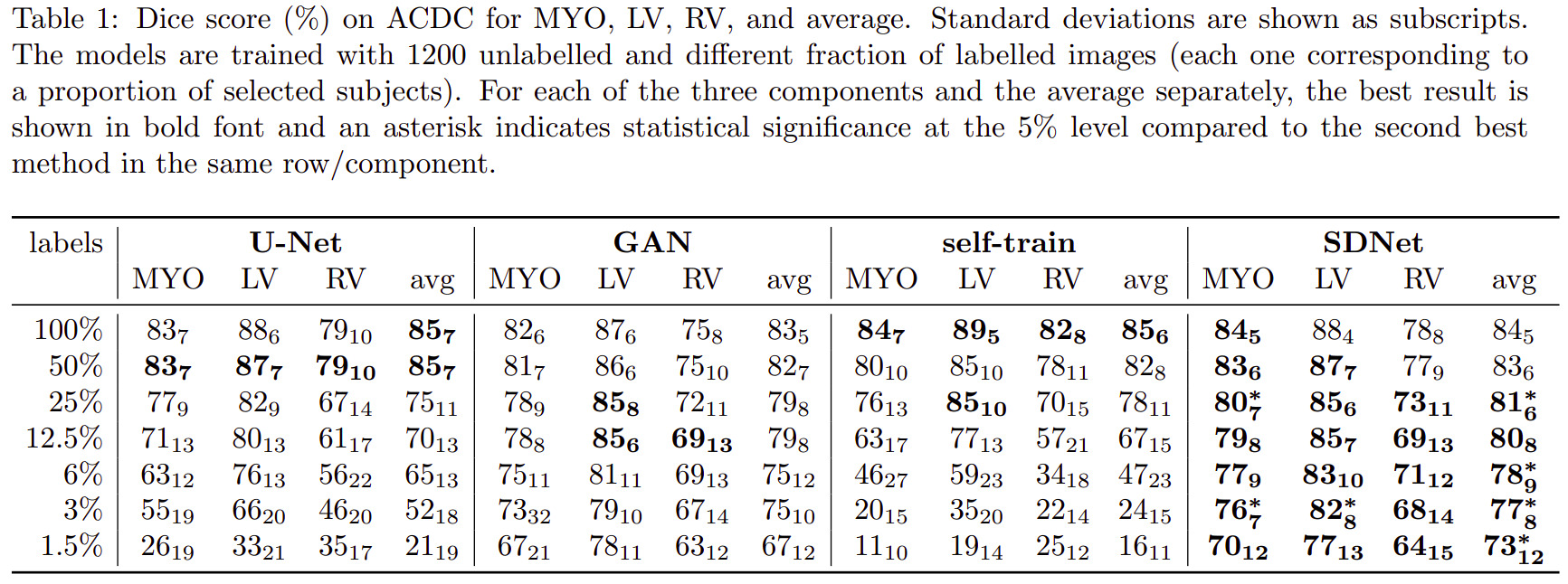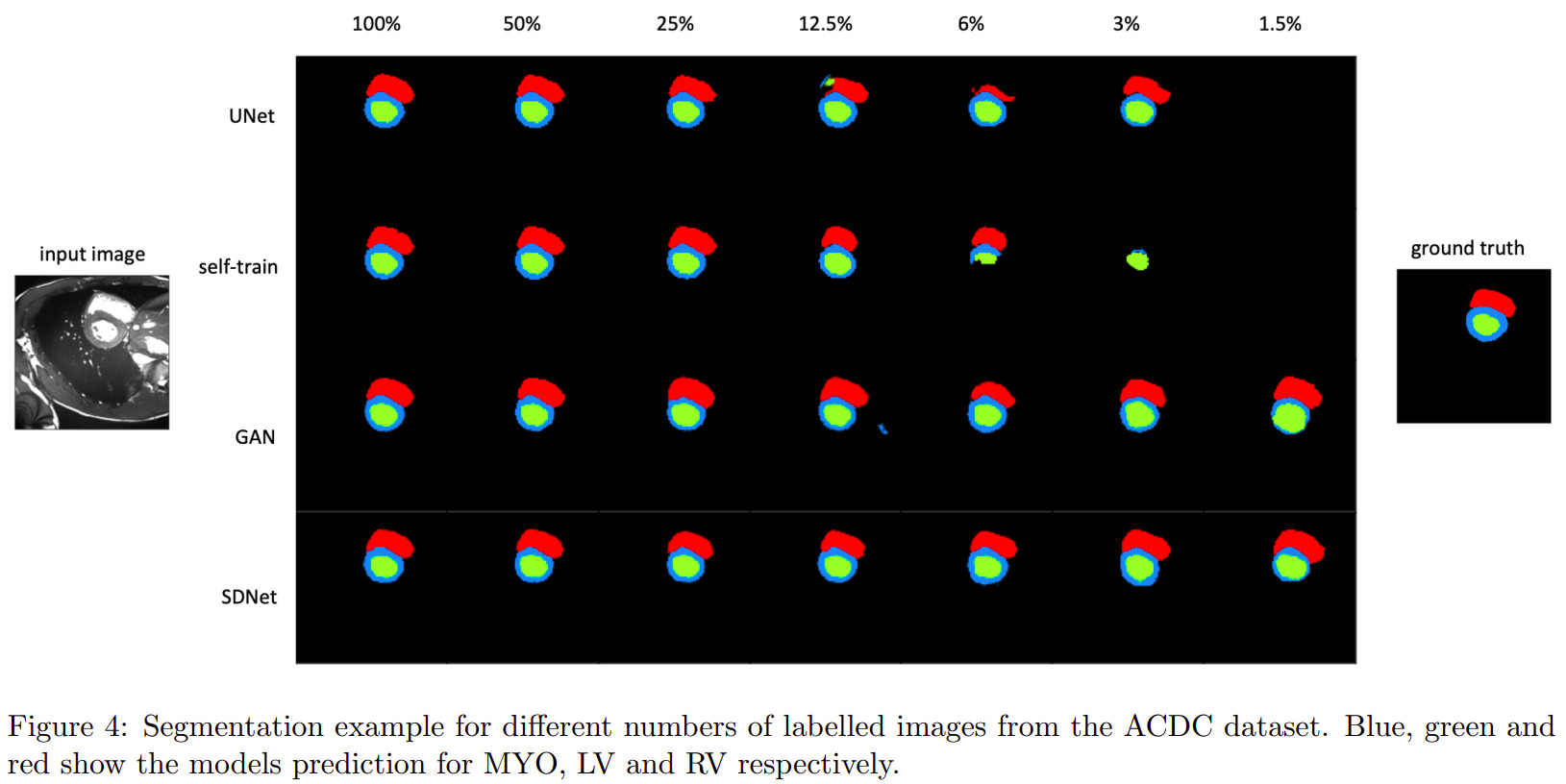## Multimodal learning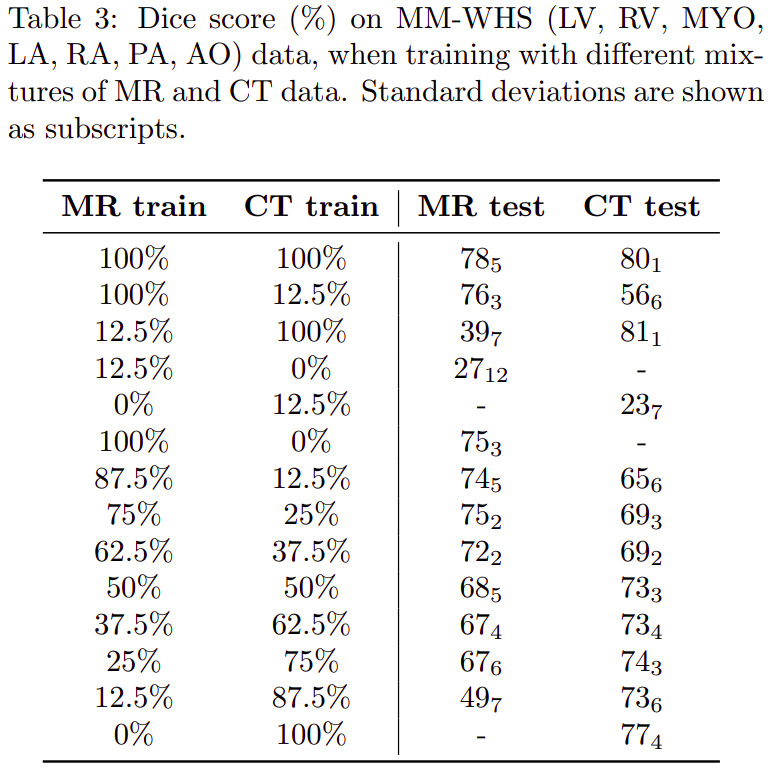## Latent space arithmetic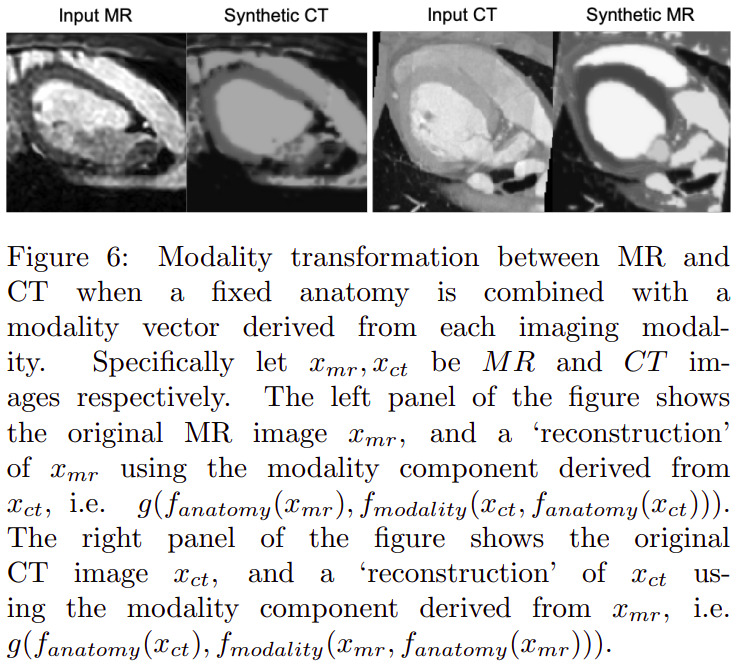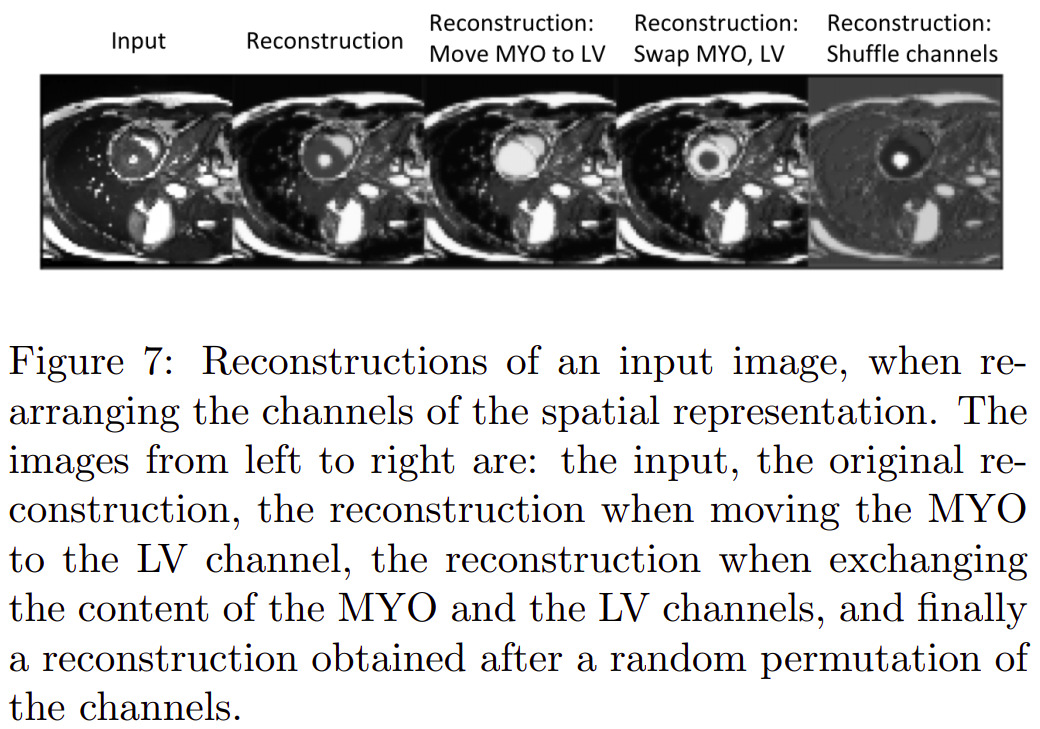## Others

Other experiments were also conducted relative to modality type estimation (from modality factors) and modality factor traversal (named factor sizes in the paper).

# Remarks

• The authors present a lot of details about their design choices, so it seems possible to reproduce accurately their experiments.